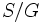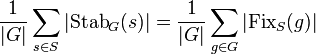# Orbit-counting theorem

Jump to: navigation, search

## Name

This result is called the orbit-counting theorem, orbit-counting lemma, Burnside's lemma, Burnside's counting theorem, and the Cauchy-Frobenius lemma.

## Statement

### In terms of group actions

Suppose a finite group$G$ has a group action on a set$S$. For$s \in S$, denote by$\operatorname{Stab}_G(s)$ the stabilizer of$s$ in$G$. Let$S/G$ be the set of orbits in$S$ under the action of$G$. Further, for$g \in G$, let$\operatorname{Fix}_S(g)$ be the set of elements of$S$ fixed by$g$. Then:$|S/G| = \frac{1}{|G|} \sum_{s \in S} |\operatorname{Stab}_G(s)| = \frac{1}{|G|} \sum_{g \in G} |\operatorname{Fix}_S(g)|$.

### In terms of linear representations

Suppose a finite group$G$ has a permutation representation on a set$S$. When viewed as a linear representation, this has character$\chi$. Then, the number of orbits of$S$ under the action of$G$ is the inner product$\langle \chi, 1_G \rangle$ where$1_G$ is the trivial (principal) character of$G$.

## Related facts

### Related counting techniques

• Polya's theorem is a more sophisticated version of this that involves a group acting on combinatorial configurations on a set.

### Related ideas in other disciplines

The formula of the orbit-counting theorem, which in this case counts the number of orbits, gives an effective measure of the size of a quotient of a set by a group action. This formula can be generalized to a groupoid acting on a set.

### Applications to conjugacy class-representation duality

The orbit-counting theorem has important applications to conjugacy class-representation duality. In particular, it is used to prove statements of the form for a given Galois automorphism group or group automorphism group, the number of orbits on the set of conjugacy classes is the same as the number of orbits on the set of irreducible representations. These statements hold even in cases where the actual orbit structures differ. (For cyclic groups of automorphisms/Galois automorphisms, the orbit structures must also coincide, by Brauer's permutation lemma).

Here are some applications:

## Proof

We first prove equality of the first two sides, and then prove equality of the second and third side.

### Equality of the first two sides

Given: A group$G$ acting on a set$S$.

To prove:$|S/G| = \frac{1}{|G|} \sum_{s \in S} |\operatorname{Stab}_G(s)|$.

Proof:

No. Assertion/construction Facts used Given data used Previous steps used Explanation
1 For any orbit$\mathcal{O}$ and any$s \in \mathcal{O}$, we have$[G:\operatorname{Stab}_G(s)] = \mathcal{O}$ Fact (1)$G$ acts on$S$ Fact-direct
2 For any orbit$\mathcal{O}$ and any$s \in \mathcal{O}$, we have$\frac{|G|}{|\operatorname{Stab}_G(s)|} = |\mathcal{O}|$ Fact (2) Step (1) [SHOW MORE]
3 For any orbit$\mathcal{O}$ and any$s \in \mathcal{O}$, we have$|\operatorname{Stab}_G(s)| = \frac{|G|}{|\mathcal{O}|}$. Step (2) Algebraic rearrangement
4 For any orbit$\mathcal{O}$, we have$\sum_{s \in \mathcal{O}} |\operatorname{Stab}_G(s)| = |G|$ Step (3) [SHOW MORE]
5 We get$\sum_{s \in S} |\operatorname{Stab}_G(s)| = |G||S/G|$. Step (4) [SHOW MORE]
6 We get$|S/G| = \frac{1}{|G|} \sum_{s \in S} |\operatorname{Stab}_G(s)|$ Step (5) Algebraic rearrangement

### Equality of the second and third side

To prove:$\frac{1}{|G|} \sum_{s \in S} |\operatorname{Stab}_G(s)| = \frac{1}{|G|} \sum_{g \in G} |\operatorname{Fix}_S(g)|$.

Proof: Note that we can ignore the$1/|G|$ on both sides for the proof. The proof essentially relies on the observation that both sides are equal to the cardinality of the set:$\{ (g,s) \in G \times S \mid g \cdot s = s \}$.

### Alternative interpretation/proof using linear representation theory

PLACEHOLDER FOR INFORMATION TO BE FILLED IN: [SHOW MORE]